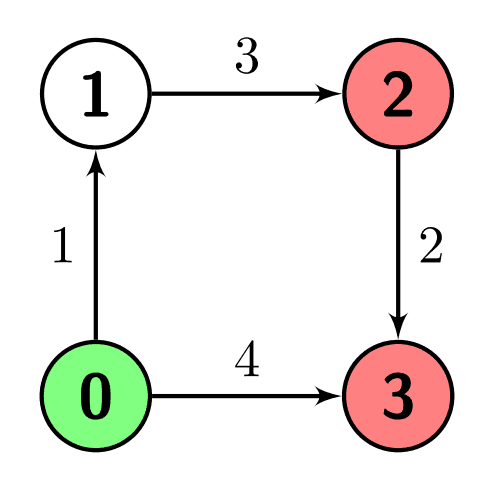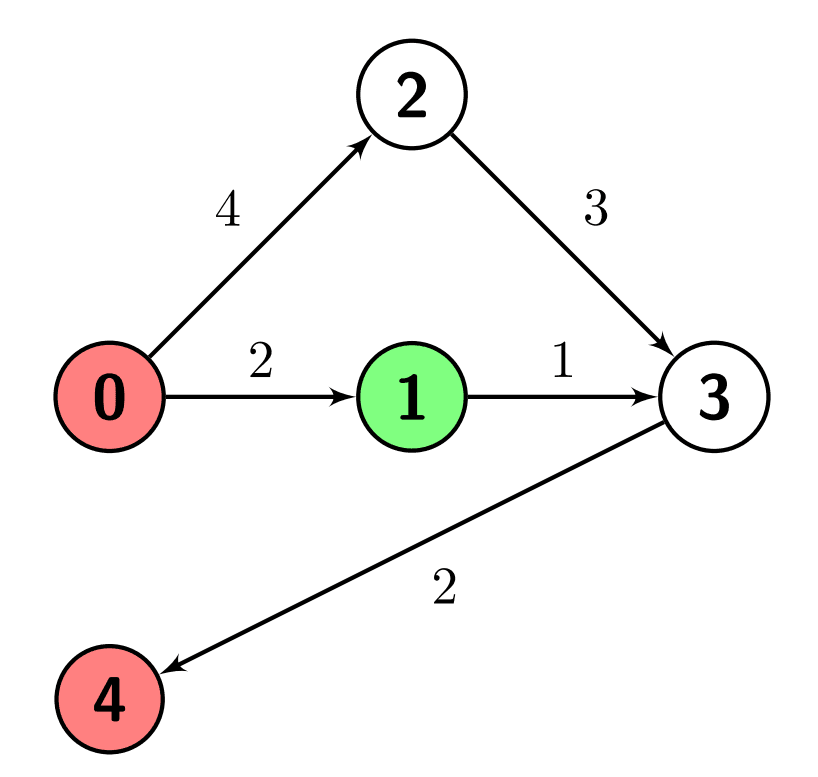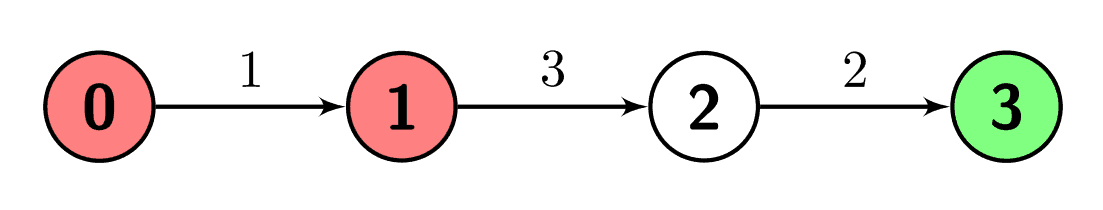# 2737. Find the Closest Marked Node

## Description

You are given a positive integer n which is the number of nodes of a 0-indexed directed weighted graph and a 0-indexed 2D array edges where edges[i] = [ui, vi, wi] indicates that there is an edge from node ui to node vi with weight wi.

You are also given a node s and a node array marked; your task is to find the minimum distance from s to any of the nodes in marked.

Return an integer denoting the minimum distance from s to any node in marked or -1 if there are no paths from s to any of the marked nodes.

Example 1:

Input: n = 4, edges = [[0,1,1],[1,2,3],[2,3,2],[0,3,4]], s = 0, marked = [2,3]
Output: 4
Explanation: There is one path from node 0 (the green node) to node 2 (a red node), which is 0->1->2, and has a distance of 1 + 3 = 4.
There are two paths from node 0 to node 3 (a red node), which are 0->1->2->3 and 0->3, the first one has a distance of 1 + 3 + 2 = 6 and the second one has a distance of 4.
The minimum of them is 4.Example 2:

Input: n = 5, edges = [[0,1,2],[0,2,4],[1,3,1],[2,3,3],[3,4,2]], s = 1, marked = [0,4]
Output: 3
Explanation: There are no paths from node 1 (the green node) to node 0 (a red node).
There is one path from node 1 to node 4 (a red node), which is 1->3->4, and has a distance of 1 + 2 = 3.Example 3:

Input: n = 4, edges = [[0,1,1],[1,2,3],[2,3,2]], s = 3, marked = [0,1]
Output: -1
Explanation: There are no paths from node 3 (the green node) to any of the marked nodes (the red nodes), so the answer is -1.Constraints:

• 2 <= n <= 500
• 1 <= edges.length <= 104
• edges[i].length = 3
• 0 <= edges[i], edges[i] <= n - 1
• 1 <= edges[i] <= 106
• 1 <= marked.length <= n - 1
• 0 <= s, marked[i] <= n - 1
• s != marked[i]
• marked[i] != marked[j] for every i != j
• The graph might have repeated edges.
• The graph is generated such that it has no self-loops.

## Solutions

• class Solution {
public int minimumDistance(int n, List<List<Integer>> edges, int s, int[] marked) {
final int inf = 1 << 29;
int[][] g = new int[n][n];
for (var e : g) {
Arrays.fill(e, inf);
}
for (var e : edges) {
int u = e.get(0), v = e.get(1), w = e.get(2);
g[u][v] = Math.min(g[u][v], w);
}
int[] dist = new int[n];
Arrays.fill(dist, inf);
dist[s] = 0;
boolean[] vis = new boolean[n];
for (int i = 0; i < n; ++i) {
int t = -1;
for (int j = 0; j < n; ++j) {
if (!vis[j] && (t == -1 || dist[t] > dist[j])) {
t = j;
}
}
vis[t] = true;
for (int j = 0; j < n; ++j) {
dist[j] = Math.min(dist[j], dist[t] + g[t][j]);
}
}
int ans = inf;
for (int i : marked) {
ans = Math.min(ans, dist[i]);
}
return ans >= inf ? -1 : ans;
}
}

• class Solution {
public:
int minimumDistance(int n, vector<vector<int>>& edges, int s, vector<int>& marked) {
const int inf = 1 << 29;
vector<vector<int>> g(n, vector<int>(n, inf));
vector<int> dist(n, inf);
dist[s] = 0;
vector<bool> vis(n);
for (auto& e : edges) {
int u = e, v = e, w = e;
g[u][v] = min(g[u][v], w);
}
for (int i = 0; i < n; ++i) {
int t = -1;
for (int j = 0; j < n; ++j) {
if (!vis[j] && (t == -1 || dist[t] > dist[j])) {
t = j;
}
}
vis[t] = true;
for (int j = 0; j < n; ++j) {
dist[j] = min(dist[j], dist[t] + g[t][j]);
}
}
int ans = inf;
for (int i : marked) {
ans = min(ans, dist[i]);
}
return ans >= inf ? -1 : ans;
}
};

• class Solution:
def minimumDistance(
self, n: int, edges: List[List[int]], s: int, marked: List[int]
) -> int:
g = [[inf] * n for _ in range(n)]
for u, v, w in edges:
g[u][v] = min(g[u][v], w)
dist = [inf] * n
vis = [False] * n
dist[s] = 0
for _ in range(n):
t = -1
for j in range(n):
if not vis[j] and (t == -1 or dist[t] > dist[j]):
t = j
vis[t] = True
for j in range(n):
dist[j] = min(dist[j], dist[t] + g[t][j])
ans = min(dist[i] for i in marked)
return -1 if ans >= inf else ans


• func minimumDistance(n int, edges [][]int, s int, marked []int) int {
const inf = 1 << 29
g := make([][]int, n)
dist := make([]int, n)
for i := range g {
g[i] = make([]int, n)
for j := range g[i] {
g[i][j] = inf
}
dist[i] = inf
}
dist[s] = 0
for _, e := range edges {
u, v, w := e, e, e
g[u][v] = min(g[u][v], w)
}
vis := make([]bool, n)
for _ = range g {
t := -1
for j := 0; j < n; j++ {
if !vis[j] && (t == -1 || dist[j] < dist[t]) {
t = j
}
}
vis[t] = true
for j := 0; j < n; j++ {
dist[j] = min(dist[j], dist[t]+g[t][j])
}
}
ans := inf
for _, i := range marked {
ans = min(ans, dist[i])
}
if ans >= inf {
return -1
}
return ans
}

func min(a, b int) int {
if a < b {
return a
}
return b
}

• function minimumDistance(n: number, edges: number[][], s: number, marked: number[]): number {
const inf = 1 << 29;
const g: number[][] = Array(n)
.fill(0)
.map(() => Array(n).fill(inf));
const dist: number[] = Array(n).fill(inf);
const vis: boolean[] = Array(n).fill(false);
for (const [u, v, w] of edges) {
g[u][v] = Math.min(g[u][v], w);
}
dist[s] = 0;
for (let i = 0; i < n; ++i) {
let t = -1;
for (let j = 0; j < n; ++j) {
if (!vis[j] && (t == -1 || dist[t] > dist[j])) {
t = j;
}
}
vis[t] = true;
for (let j = 0; j < n; ++j) {
dist[j] = Math.min(dist[j], dist[t] + g[t][j]);
}
}
let ans = inf;
for (const i of marked) {
ans = Math.min(ans, dist[i]);
}
return ans >= inf ? -1 : ans;
}